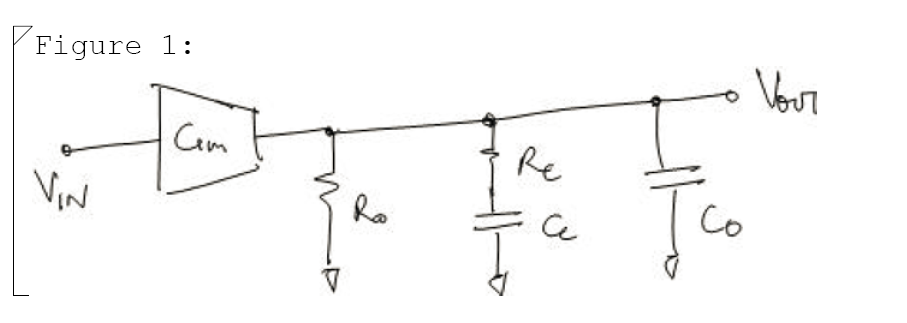# Circuit [top]# Equations [top]

`\$\$V_{out}={G_mV_{in}/{C_os+1/{1/{C_cs}+R_c}+1/R_o}\$\$`
or
`\$\$V_{out}/V_{in} = {C_cG_mR_cR_os+G_mR_o}/{C_cC_oR_cR_os^2+((C_o+C_c)R_o+C_cR_c)s+1}..(1)\$\$`

# Poles and Zeroes [top]

The zero is simply
`\$\$ s = -1/{C_cR_c}\$\$`

The poles can be fully evaluated as done here to get the following values:

`\$\$p_1={-(C_O+C_C)R_O-R_CC_C-√{C_C^2R_C^2+2C_CR_OR_C(C_C-C_O)+(C_C+C_O)^2R_O^2}}/{2C_CC_OR_OR_C}\$\$`

`\$\$p_2={-(C_O+C_C)R_O-R_CC_C+√{C_C^2R_C^2+2C_CR_OR_C(C_C-C_O)+(C_C+C_O)^2R_O^2}}/{2C_CC_OR_OR_C}\$\$`

We can further simplify the pole expressions to more intuitive ones for design. If we substitute the following:
`\$\$A=C_cC_oR_cR_o\$\$`

`\$\$B=(C_o+C_c)R_o+C_cR_c\$\$`

we can write (1) as:
`\$\$As^2+Bs+1=0\$\$`

We can solve for s as:
`\$\$s={-B±√{B^2-4A} }/{2A}\$\$`

`\$\$s=-B/{2A}±B/{2A}√{1-{4A}/B^2}\$\$`

Now to simplify further we need to see the relative magnitudes to decide what terms can be neglected. Comparing A and B we can clearly see that if the circuit time constants are lower than even a milli second A ≪ B. That is because A is a product of 2 time constants while B is a time constant. Usually the network is designed to have 1 time constant much smaller than the other. So consider the case when one time constant is 100 times larger than the other. For example `\$R_cC_c ≫ R_oC_o\$`. This means that the magnitude of `\$A/B^2\$` = 100/10000 or `\$4A/B^2=4/100\$`. So `\$B^2\$` is still 25 times larger than 4A.

So from this point on the assumption and approximation that we take is:

One time constant in the circuit is more dominant than the other one.

So now we can approximate the poles now as:
`\$\$s≈-B/{2A}±B/{2A}(1-{2A}/B^2)\$\$`

`\$\$p_1≈-1/B ; p_2≈-B/A\$\$`

So substituting the values of A and B in we get the final poles of the circuit as:
`\$\$p_1≈-1/{(C_o+C_c)R_o+C_cR_c}\$\$`

`\$\$p_2≈-{(C_o+C_c)R_o+C_cR_c}/{C_cC_oR_cR_o}\$\$`

2. The book Frequency Compensation Techniques for Low-Power Operational Amplifiers (The Springer International Series in Engineering and Computer Science)has good chapter on compensation analysis of multi stage opamps.
3. The book Op Amps for Everyone, Fourth Editionhas a good chapter on different networks for compensating opamps